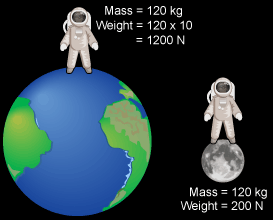A# weightWeight is the force exerted on an object with mass by a gravitational field, such as that of a planet. Weight is experienced as a reaction to this force against a solid surface. Although the mass of an object is independent of location, its weight varies depending on the strength of the local gravitational field.

Weight is given by the product of mass (m) and the acceleration due to gravity (g), that is: W = mg. Use the table below to figure out how much you woulld weigh on another planet of the solar system or the Moon: simply multiply your weight by the conversion factor. (Note: The gas giants, Jupiter, Saturn, Uranus, Neptune, don't have solid surfaces, so the values shown pretend that they do.)

Weight on other worlds
Place Conversion factor Weight of a person whose
mass is 60 kg (132 lb) on Earth
Moon × 0.166 99.6 N
Mercury × 0.378 226.8 N
Venus × 0.907 544.2 N
Earth - 600 N
Mars × 0.377 226.2 N
Jupiter × 2.36 1416.0 N
Saturn × 0.916 549.6 N
Uranus × 0.889 533.4 N
Neptune × 1.12 672.0 N
Pluto × 0.059 35.4 N